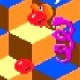# The words “Maurice Bernard Sendak”.Note: Translated words like ‘Maurice Bernard Sendak’ indicate unusual relationships between the character positions in the word(s) being translated and result of the translation. God (UFO/ET) talks to us through the ET Corn Gods language translation of words.
Maurice Bernard Sendak
Words and groups of words are like computer programs which explode into a family of predetermined meanings.

Maurice Bernard Sendak:
Maurice:
Maurice = eciram
Ec+66+66 ,.. = “re”.
R+e is “W”.
I is 9, ix, ix-66-66 … = “0”, drop it.
Ra is r+a, S, S+66 = “He”.
Therefore:
Maurice = “Whe M”
E is 5, 55, 555.  (e is five, I-f ve = c ve = ev, again eve.
E is 5, 50, L, 12, 12+66+66 … = “Nd”, n+d = R.
Therefore:
Maurice = “Where M”
1+0 = 10, 10+66+66 = “Th”.
Therefore:
Maurice = “Where the m”
E is five, eeee, (see above)
E is 5, 2+3, “23”, “W”.
Add 0+66+66 … = “924”, nine, “I”.
E is 5, 50, L, 500, D.
Therefore:
Maurice = “Where the Wild M”
M is ac, book of acts = “S”.  (act-66-66 .. = “0”, drop it)
E is 5, 5 is five, I-f ve = “ve”, ee.
E + 0 = 50, L, 12+66+66 .. = Nd, N+D, R.
Therefore:
Maurice = “Where the Wild Things Are”
Bernard:
B:
Add 0+66+66 .. = “or”, backwards is Ro, book 45, backwards = 54
Therefore:
Therefore:
Bernard = “Dead May 8 arnard”.
Ar-66-66 .. = “52”, book Ath.
At is at-66, 54, backwards is 45, book Ro, backwards is or, or-66-66 .. = “9”, drop it.
H is 80, 80-66 = “14”, 2+12, “2012”.
Therefore:
Ard = aa hd.
Aa+66+66+66 … = “GRS”, g is b+e, “Y”
Add o, 15, backwards is 51, ea
Therefore:
Sh-66-66 .. = “0”, drop it.
D is 500, 5, book Dt, t is 20, bo, b+15, ag, g+66+66  =  Te
Te is 20+5, “Y”.
Therefore:
Sendak:
E is 5, book dt, d+t = “24”, book Jer, 24+56, 80. (j is 10, x, 24. Er-66-66 . = “56”)
80-66 = “14”, 1 22, 2 is B, Boron = “Bn”. (or-66-66 .. = “0”, drop “o”)
Therefore:
14 = 1414, 28.
Therefore:
D is 4, 2+2.
2 is B, Boron, “Born”. (Drop “o”)
Therefore:
Sendak = “1928 AD, Born bak”.
B is Born, “Bad”, 16, 10+6, June 10.
ak = is 111, ck = “K”.
K+66+66 … = “GRS”.
G is 7, 2+5, Y.
Add o, 15, backwards is 51.
Therefore:
K = “Year”.s.
S is 19, one I
E is 5, book Dt, “Day”. (t is 20, bo, b+o, ag, g+66+66 .. = “te”, 20+5, “Y”)
I is 9, ix, 924-66-66 … = “0”, drop it.
Therefore:
Sendak = “1928 AD, June 10, Born Year On Day”.

\Visit:

## 7 thoughts on “The words “Maurice Bernard Sendak”.”

1. Pingback: The word “Event”. | UfoEtBlog.com

2.Tim H. on said:

Fascinating. So who’s going to die next?

•admin on said:

Thanks for asking. I can’t predict. Only after the Death, you can see that the date of death (and birth) was pre-coded into the name of the person. Scary stuff. everything is Predetermined.

The word “Predetermined”.
Predermined:
e is five, i-f ve = “ee”.
et is 52, book ath.
Therefore:
Predetermined = “Pre death ermined:
e is five, i-f ve = cve, ee.
e is 5, book “Dt”, “Date”. (t is bo, b+o, 17, 7+66+66 .. = “Te”)
r is N+D, nd, nd-66-66 = “L”.
e+66 = “Ga”.
Gal = Gr, gr-66-66 .. = “ET”.
mi-66-66 = “G”.
ne+66+66 .. = “Me”.
ed is 45, backwards, book Romans, backwards is “Snamor”.
Sn is 19 AD, book 58, 58+66+66 … = “GR”.
a+66+66 = “Si”, add 0+66+66 … = “Sn”, “Son”, “Pson”. (add “o”, s is 19, book PS)
Therefore:
Predetermined = “Pre Death Date ET Game G R Simpson”.

3.Tim H. on said:

If you can only see the connection afterwards, how do you know it’s pre-determined?

4.admin on said:

You can find that date, and perhaps the birth date, but not other dates. Think about it.

5.Tim H. on said:

I just found out about this, so I’m no expert. So let me put it this way:

In two days, it will be Monday, May 14, 2012. Are there any celebrities who definitely will not die that day, because it is not encoded in their name?